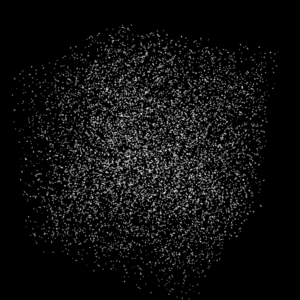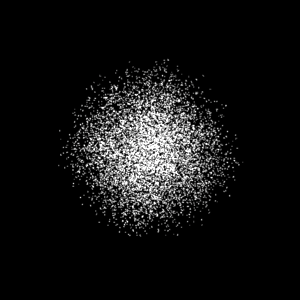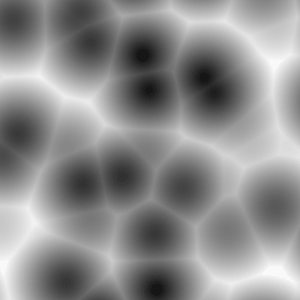### RSLNebula Point Clouds

#### Introduction

This page presents a technique for generating a point cloud that mimics the sinuous distribution of stars embedded within a nebula - figure 1. The shader shown in listing 2 is similiar to the one used to bake a 3D sierpinski gasket.

Because the shader is used on a single point primitive we can be sure it will only be called once. For that reason the shader can perform calculations that otherwise would be prohibitively expensive.

The shader consists of a loop within which,

1. a random point is generated,
2. the point is offset by -0.5, -0.5, -0.5,
3. the point is used to define a vector,
4. the vector is normalized,
5. and its length randomized,
6. a density function calculates a value that determines whether or not the coordinates of the vector should be baked into a point cloud.

The calculations within the loop are visualized in figures 2 to 5.Figure 2 - Random pointsFigure 3 - Points on a sphereFigure 4 - Points in a sphereFigure 5 - Output from worley()

Listing 1 (density.h)

 ```// From pages 257 and 258 of Advanced RenderMan by Apodaca & Gritz float worley(point p; string spacename; float freq) { point pp = transform(spacename, p) * freq; point thiscell = point(floor(xcomp(pp))+0.5, floor(ycomp(pp))+0.5, floor(zcomp(pp))+0.5); float dist2nearest = 1000; uniform float i,j,k; for(i = -1; i <= 1; i+= 1) for(j = -1; j <= 1; j+= 1) for(k = -1; k <= 1; k+= 1) { point testcell = thiscell + vector(i,j,k); point pos = testcell + vector cellnoise(testcell)-0.5; float dist = distance(pos,pp); if(dist < dist2nearest) dist2nearest = dist; } return dist2nearest; }```

Listing 2

 ```#include "density.h" surface nebula(float iterations = 100000, mindensity = 0.8; string bakename = "") { normal n = normal(0,0,0); float j, x, y, z; point p; vector vec; float density; for(j = 0; j < iterations; j = j + 1) { // Generate a random point p = random(); p = (p - 0.5) * 2; vec = vector "world" (xcomp(p), ycomp(p), zcomp(p)); // Randomize the length of the vector so // that we get locations distributed more // or less uniformly within the sphere. vec = normalize(vec) * random(); p = point "world" (xcomp(vec), ycomp(vec), zcomp(vec)); // Generate a value based on our point position density = worley(p,"object", 5); if(density >= mindensity) { bake3d(bakename, "P,N,Os", p, n, "P", p, "N", n, "Os", color(density,density,density)); } } Oi = Cs; Ci = Oi * Cs; }```

#### Bake Pass/Beauty Pass

Listing 3 provides a sample rib file suitable for baking a nebula point cloud. Listing 4 provides a beauty pass sample rib file for rendering the nebula brickmap as geometry.

Listing 3 (bake pass)

 ```DisplayChannel "color P" DisplayChannel "color N" DisplayChannel "color Os" Display "nebula" "framebuffer" "rgba" Format 800 800 1 Projection "perspective" "fov" 40 ShadingRate .01 LightSource "distantlight" 1 "intensity" 1.5 "from" [0 0 0] "to" [0 0 1] Translate 0 0 4 Rotate -10 1 0 0 Rotate 0 0 1 0 Scale 1 1 -1 WorldBegin TransformBegin Surface "nebula2" "mindensity" 0.8 "bakename" ["./nebula.ptc"] "iterations" 1000000 Points "P" [0 0 0] "constantwidth" [0.008] TransformEnd WorldEnd System "brickmake ./nebula.ptc ./nebula.bkm"```

Listing 4 (beauty pass)

 ```Display "nebula" "framebuffer" "rgba" Format 400 400 1 Projection "perspective" "fov" 30 ShadingRate 20 Translate 0 0 4 Rotate -10 1 0 0 Rotate 0 0 1 0 Scale 1 1 -1 WorldBegin LightSource "pointlight" 2 "intensity" 0.25 "from" [0 0.6 0] "lightcolor" [0.5 0.5 1] LightSource "pointlight" 3 "intensity" 0.25 "from" [-0.4 -0.6 0] "lightcolor" [1 0.25 0.25] LightSource "pointlight" 4 "intensity" 5 "from" [3 0 1] "lightcolor" [1 0.85 1] AttributeBegin Surface "nebula_show". Geometry "brickmap" "filename" "./nebula.bkm" AttributeEnd WorldEnd```

Listing 5 gives the code for a shader that considers the normal of the micro-polygon being shaded to face the incident ray ie. the viewing vector. This ensures that when the brickmap is rendered as geometry all parts of the nebula have equal brightness. It also uses a function that can apply a noisey offset to the normal and, hence, to the brightness of the micro-polygon.

Listing 5

 ```vector wobble(point p; float amp) { vector result = noise(p * 4) * amp; return result; } //------------------------------ surface nebula_show(float Kd = 1) { normal fakeN = normalize(-I); fakeN += wobble(transform("shader", P), 1.8); color diffusecolor = Kd * diffuse(fakeN); Oi = Os; Ci = Oi * Cs * diffusecolor; }```